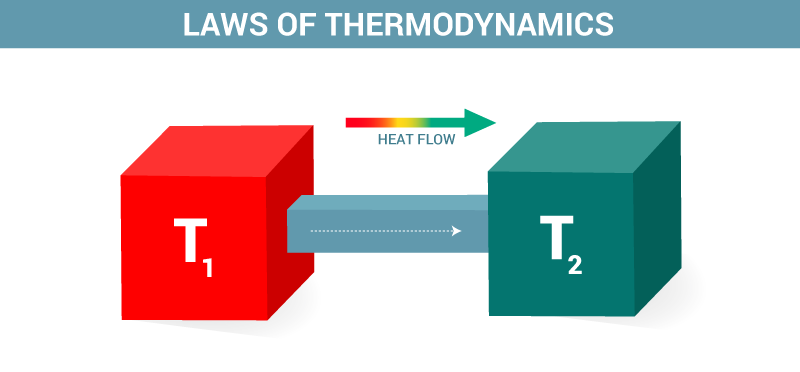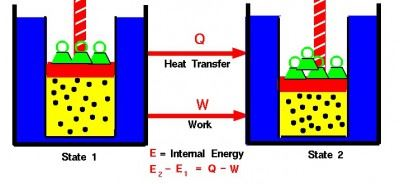# First Law Of Thermodynamics and Energy Conservation## What is the first law of thermodynamics?

We know about internal energy, heat, and work done for a system in thermodynamics, but how are these quantities related to each other. The laws of thermodynamics, give us the guidelines for inter-conversion of energy from one form to the other. The history of thermodynamics dates back to the time when first heat engines were designed for the extraction of water from mines. The studies for improving the efficiency and output of these engines continued for a longer time. The first law of thermodynamics was conceived by a trial and error method that stretched over a period of fifty years.

### First law of thermodynamics statement:

The thermodynamics first law states that ” The total energy of an isolated system is constant.”

A close examination of this statement suggests that the first law of thermodynamics is nothing but conservation of energy. The basis for the development of this law is the conservation of energy. As per the thermodynamic approach, this law is stated as the internal energy change of any closed system is given by the difference between the heat stored in a system and the work done by it. An equivalent way of stating the first law of thermodynamics is that perpetual motion machines, which can indefinitely do work without any energy source are impossible as it violates the conservation of energy and thermodynamics first law.

To learn in details about all the laws of thermodynamics visit the links given below.

### Mathematical Representation:Mathematically the first law of Thermodynamics is stated as:

$~~~~~~~~~~~~~~~~$ ΔU = Q – W

Where ΔU is the change in internal energy of the system.

Q is the heat given to the system.

W is work done by the system.

Internal energy is a state function and is measured as the change in its absolute value, which cannot be measured and hence holds no value in absolute terms. The heat given to the system is always taken positive and the work done by the system is also positive. If heat is being extracted (given out) from the system then it is taken as negative and any work done on the system is also taken negatively while calculating thermodynamic properties.

The laws of thermodynamics played a vital role in designing various engines and optimizing their efficiency and output. To know more about the laws of thermodynamics, download Byju’s The Learning App.